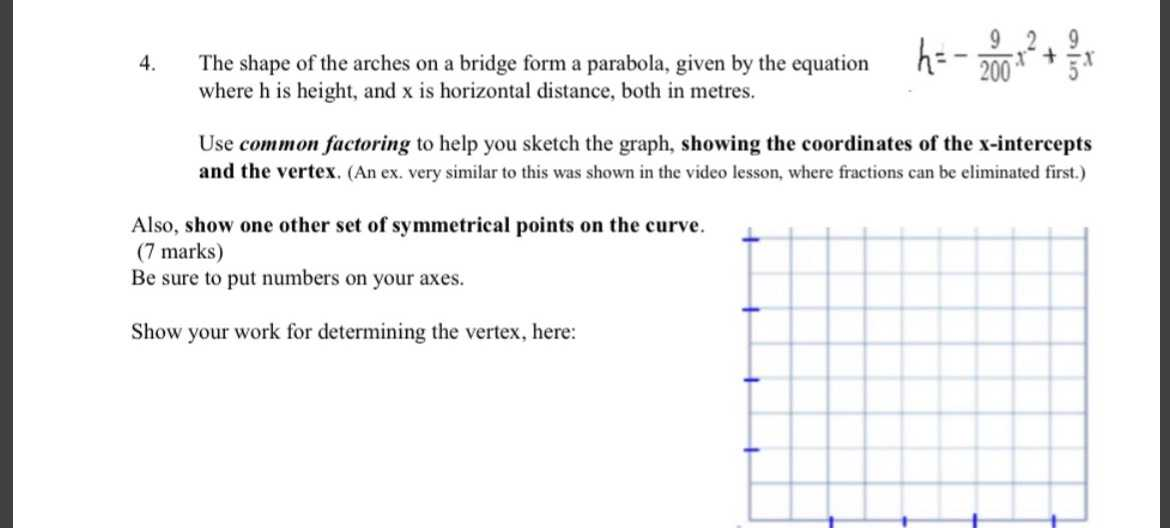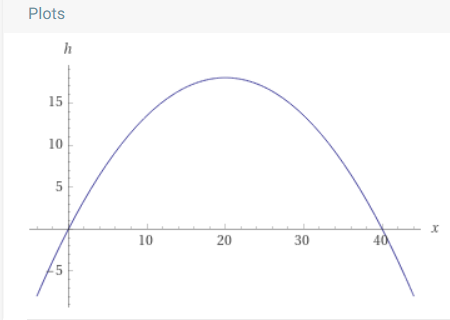### ¿Todavía tienes preguntas de matemáticas?

Pregunte a nuestros tutores expertos
Algebra
Pregunta4. The shape of the arches on a bridge form a parabola, given by the equation $$h = - \frac { 9 } { 200 } x ^ { 2 } + \frac { 9 } { 5 } x$$

where h is height, and $$x$$ is horizontal distance, both in metres.

Use common factoring to help you sketch the graph, showing the coordinates of the x-intercepts and the vertex. (An ex. very similar to this was shown in the video lesson, where fractions can be eliminated first.)

Also, show one other set of symmetrical points on the curve. ( $$7$$ marks)

Be sure to put numbers on your axes. Show your work for determining the vertex, here: